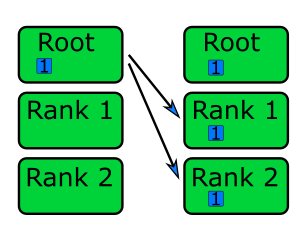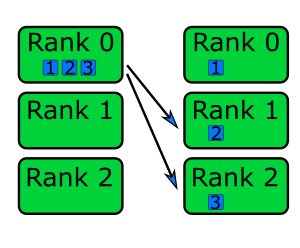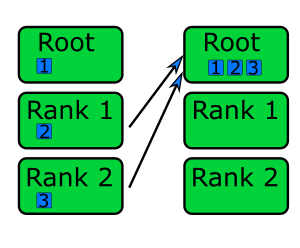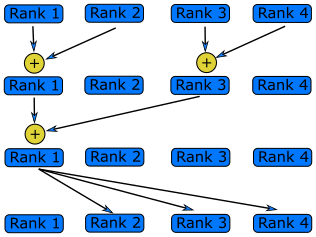This lesson is being piloted (Beta version)

# Collective Operations

## Overview

Teaching: 10 min
Exercises: 20 min
Questions
• What other useful functions does MPI have?

Objectives
• Introduce collective operations.

Display Language

## Collective Operations

There are several special cases that are implemented in the MPI standard. The most commonly-used are:

• Synchronisation
• Wait until all processes have reached the same point in the program.
• MPI_Barrier().
• Mainly useful for debugging and solving timing related problems.
• One-To-All Communication:
• One rank sends the same message to all other ranks.
• MPI_Bcast(), MPI_Scatter().
• Useful for sending input or commands to all other ranks.
• All-to-One
• All ranks send data to a single rank.
• MPI_Reduce(), MPI_Gather().
• All-to-All
• All ranks have data and all ranks receive data.
• Global reductions is an important special case.
• MPI_Alltoall(), MPI_Allgather().
• MPI_Allreduce().

### Barrier

 int MPI_Barrier( MPI_Comm communicator )

 MPI_Barrier(COMM)
INTEGER    COMM

def barrier(self)


Wait (doing nothing) until all ranks have reached this line.

### Broadcast

 int MPI_Bcast(
void* data,
int count,
MPI_Datatype datatype,
int root,
MPI_Comm communicator)

MPI_Bcast(buffer, count, datatype, root, COMM, IERROR)
<type>    buffer(*)
INTEGER    count, datatype, root, COMM, IERROR

def bcast(self, obj, int root=0)Very similar to MPI_Send, but the same data is sent from rank root to all ranks. This function will only return once all processes have reached it, meaning it has the side-effect of acting as a barrier.

### Scatter

 int MPI_Scatter(
void* sendbuf,
int sendcount,
MPI_Datatype sendtype,
void* recvbuffer,
int recvcount,
MPI_Datatype recvtype,
int root,
MPI_Comm communicator)

MPI_Scatter(sendbuf, sendcount, sendtype, recvbuffer, recvcount,
recvcount, root, COMM, IERROR)
<type>    sendbuf(*), recvbuf(*)
INTEGER    sendcount, sendtype, recvcount, recvtype, root
INTEGER    COMM, IERROR

def scatter(self, sendobj, int root=0)The data in the sendbuf on rank root is split into chunks and each chunk is sent to a different rank. Each chunk contains sendcount elements of type sendtype. So if sendtype is MPI_Int, and sendcount is 2, each rank will receive 2 integers. The received data is written to the recvbuf, so the sendbuf is only needed by the root. The next two parameters, recvcount and recvtype describe the receive buffer. Usually recvtype is the same as sendtype and recvcount is Nranks*sendcount.

### Gather

int MPI_Gather(
void* sendbuf,
int sendcount,
MPI_Datatype sendtype,
void* recvbuffer,
int sendcount,
MPI_Datatype recvtype,
int root,
MPI_Comm communicator)

MPI_Gather(sendbuf, sendcount, sendtype, recvbuf, recvcount,
recvtype, root, COMM, IERROR)
<type>    sendbuf(*), recvbuf(*)
INTEGER    sendcount, sendtype, recvcount, recvtype, root
INTEGER    COMM, IERROR

def gather(self, sendobj, int root=0)Each rank sends the data in the sendbuf to rank root. The root collects the data into the recvbuffer in order of the rank numbers.

## Sending and Receiving

In the morning we wrote a hello world program where each rank sends a message to rank 0. Write this using a gather instead of send and receive.

## Solution

#include <stdio.h>
#include <stdlib.h>
#include <mpi.h>

int main(int argc, char** argv) {
int rank, n_ranks, numbers_per_rank;
char send_message, *receive_message;

// First call MPI_Init
MPI_Init(&argc, &argv);
// Get my rank and the number of ranks
MPI_Comm_rank(MPI_COMM_WORLD, &rank);
MPI_Comm_size(MPI_COMM_WORLD, &n_ranks);

// Allocate space for all received messages in receive_message
receive_message = malloc( n_ranks*40*sizeof(char) );

//Use gather to send all messages to rank 0
sprintf(send_message, "Hello World, I'm rank %d\n", rank);
MPI_Gather( send_message, 40, MPI_CHAR, receive_message, 40, MPI_CHAR, 0, MPI_COMM_WORLD );

if(rank == 0){
for( int i=0; i<n_ranks; i++){
printf("%s", receive_message + i*40);
}
}

// Free memory and finalise
free( receive_message );
return MPI_Finalize();
}


## Solution

program hello

implicit none
include "mpif.h"

integer n_ranks, rank, sender, ierr
character(len=40) send_message
character, dimension(:), allocatable :: receive_message

! First call MPI_Init
call MPI_Init(ierr)

! Get my rank and the number of ranks
call MPI_Comm_rank(MPI_COMM_WORLD, rank, ierr)
call MPI_Comm_size(MPI_COMM_WORLD, n_ranks, ierr)

! Allocate space for all received messages in receive_message
allocate ( receive_message(n_ranks*40) )

! Use gather to send all messages to rank 0
write(send_message,*) "Hello World, I'm rank", rank
call MPI_Gather( send_message, 40, MPI_CHARACTER, receive_message, 40, MPI_CHARACTER, 0, MPI_COMM_WORLD, ierr )

if (rank == 0) then
do sender = 1, n_ranks-1
write(6,*) receive_message(40*sender: 40*(sender+1))
end do
end if

! Free memory and finalise
deallocate( receive_message )
call MPI_Finalize(ierr)
end


## Solution

from mpi4py import MPI

# Get my rank and the number of ranks
rank = MPI.COMM_WORLD.Get_rank()
n_ranks = MPI.COMM_WORLD.Get_size()

# Use gather to send all messages to rank 0
send_message = "Hello World, I'm rank {:d}".format(rank)
receive_message = MPI.COMM_WORLD.gather(send_message, root=0)

if rank == 0:
for i in range(n_ranks):
print(receive_message[i])


### Reduce

int MPI_Reduce(
void* sendbuf,
void* recvbuffer,
int count,
MPI_Datatype datatype,
MPI_Op op,
int root,
MPI_Comm communicator)

MPI_Reduce(sendbuf, recvbuf, count, datatype, op, root, COMM,
IERROR)
<type>    sendbuf(*), recvbuf(*)
INTEGER    count, datatype, op, root, COMM, IERROR

def reduce(self, sendobj, op=SUM, int root=0)Each rank sends a piece of data, which are combined on their way to rank root into a single piece of data. For example, the function can calculate the sum of numbers distributed across all the ranks.

Possible operations include:

• MPI_SUM: Calculate the sum of numbers sent by each rank.
• MPI_MAX: Return the maximum value of numbers sent by each rank.
• MPI_MIN: Return the minimum of numbers sent by each rank.
• MPI_PROD: Calculate the product of numbers sent by each rank.
• MPI_MAXLOC: Return the maximum value and the number of the rank that sent the maximum value.
• MPI_MINLOC: Return the minimum value of the number of the rank that sent the minimum value.

In Python, these operations are named MPI.SUM, MPI.MAX, MPI.MIN, and so on.

The MPI_Reduce operation is usually faster than what you might write by hand. It can apply different algorithms depending on the system it’s running on to reach the best possible performance. This is particularly the case on systems designed for high performance computing, where the MPI_Reduce operations can use the communication devices to perform reductions en route, without using any of the ranks to do the calculation.

### Allreduce

int MPI_Allreduce(
void* sendbuf,
void* recvbuffer,
int count,
MPI_Datatype datatype,
MPI_Op op,
MPI_Comm communicator)

MPI_Allreduce(sendbuf, recvbuf, count, datatype, op, COMM, IERROR)
<type>    sendbuf(*), recvbuf(*)
INTEGER    count, datatype, op, COMM, IERROR

def allreduce(self, sendobj, op=SUM)MPI_Allreduce performs essentially the same operations as MPI_Reduce, but the result is sent to all the ranks.

## Reductions

The following program creates an array called vector that contains a list of n_numbers on each rank. The first rank contains the numbers from 1 to n_numbers, the second rank from n_numbers to 2*n_numbers2 and so on. It then calls the find_max and find_sum functions that should calculate the sum and maximum of the vector.

These functions are not implemented in parallel and only return the sum and the maximum of the local vectors. Modify the find_sum and find_max functions to work correctly in parallel using MPI_Reduce or MPI_Allreduce.

#include <stdio.h>
#include <mpi.h>

// Calculate the sum of numbers in a vector
double find_sum( double * vector, int N ){
double sum = 0;
for( int i=0; i<N; i++){
sum += vector[i];
}
return sum;
}

// Find the maximum of numbers in a vector
double find_maximum( double * vector, int N ){
double max = 0;
for( int i=0; i<N; i++){
if( vector[i] > max ){
max = vector[i];
}
}
return max;
}

int main(int argc, char** argv) {
int n_numbers = 1024;
int rank;
double vector[n_numbers];
double sum, max;
double my_first_number;

// First call MPI_Init
MPI_Init(&argc, &argv);

// Get my rank
MPI_Comm_rank(MPI_COMM_WORLD, &rank);

// Each rank will have n_numbers numbers,
// starting from where the previous left off
my_first_number = n_numbers*rank;

// Generate a vector
for( int i=0; i<n_numbers; i++){
vector[i] = my_first_number + i;
}

//Find the sum and print
sum = find_sum( vector, n_numbers );
printf("The sum of the numbers is %f\n", sum);

//Find the maximum and print
max = find_maximum( vector, n_numbers );
printf("The largest number is %f\n", max);

// Call finalize at the end
return MPI_Finalize();
}

program sum_and_max

implicit none
include "mpif.h"

integer rank, n_ranks, ierr

integer, parameter :: n_numbers=10
real vector(n_numbers)
real vsum, vmax, my_first_number
integer i

! First call MPI_Init
call MPI_Init(ierr)

! Get my rank and the number of ranks
call MPI_Comm_rank(MPI_COMM_WORLD, rank, ierr)

! Each rank will have n_numbers numbers,
! starting from where the previous left
my_first_number = n_numbers*rank;

! Set the vector
do i = 1, n_numbers
vector(i) = my_first_number + i
end do

! Find the sum and print
call find_sum( vector, n_numbers, vsum )
write(6,*) "Sum = ", vsum

! Find the maximum and print
call find_max( vector, n_numbers, vmax )
write(6,*) "Maximum = ", vmax

! Call MPI_Finalize at the end
call MPI_Finalize(ierr)

contains

! Calculate the sum of numbers in a vector
subroutine find_sum( vector, N, vsum )
real, intent(in) :: vector(:)
real, intent(inout) :: vsum
integer, intent(in) :: N
integer i

vsum = 0
do i = 1, N
vsum = vsum + vector(i)
end do

end subroutine find_sum

! Find the maximum of numbers in a vector
subroutine find_max( vector, N, vmax )
real, intent(in) :: vector(:)
real, intent(inout) :: vmax
integer, intent(in) :: N
integer i

vmax = 0
do i = 1, N
if (vmax < vector(i)) then
vmax = vector(i)
end if
end do

end subroutine find_max
end

from mpi4py import MPI

# Calculate the sum of numbers in a vector
def find_sum(vector):
my_sum = 0.0
for i in range(len(vector)):
my_sum += vector[i]
return my_sum

# Find the maximum of numbers in a vector
def find_maximum(vector):
my_max = 0.0
for i in range(len(vector)):
if vector[i] > my_max:
my_max = vector[i]
return my_max

n_numbers = 1024

# Get my rank
rank = MPI.COMM_WORLD.Get_rank()

# Each rank will have n_numbers numbers,
# starting from where the previous left off
my_first_number = n_numbers*rank

# Generate a vector
vector = []
for i in range(n_numbers):
vector.append(float(my_first_number + i))

# Find the sum and print
my_sum = find_sum(vector)
print("The sum of the numbers is", my_sum)

# Find the maximum and print
my_max = find_maximum(vector)
print("The largest number is", my_max)


## Solution

// Calculate the sum of numbers in a vector
double find_sum( double * vector, int N ){
double sum = 0;
double global_sum;

// Calculate the sum on this rank as before
for( int i=0; i<N; i++){
sum += vector[i];
}

// Call MPI_Allreduce to find the full sum
MPI_Allreduce( &sum, &global_sum, 1, MPI_DOUBLE, MPI_SUM, MPI_COMM_WORLD );

return global_sum;
}

// Find the maximum of numbers in a vector
double find_maximum( double * vector, int N ){
double max = 0;
double global_max;

// Calculate the sum on this rank as before
for( int i=0; i<N; i++){
if( vector[i] > max ){
max = vector[i];
}
}

// Call MPI_Allreduce to find the maximum over all the ranks
MPI_Allreduce( &max, &global_max, 1, MPI_DOUBLE, MPI_MAX, MPI_COMM_WORLD );

return global_max;
}


## Solution

contains

! Calculate the sum of numbers in a vector
subroutine find_sum( vector, N, global_sum )
implicit none
include "mpif.h"

real, intent(in) :: vector(:)
real, intent(inout) :: global_sum
real vsum
integer, intent(in) :: N
integer i, ierr

vsum = 0
do i = 1, N
vsum = vsum + vector(i)
end do

! Call MPI_Allreduce to find the full sum
call MPI_Allreduce( vsum, global_sum, 1, MPI_REAL, MPI_SUM, MPI_COMM_WORLD, ierr )

end subroutine find_sum

! Find the maximum of numbers in a vector
subroutine find_max( vector, N, global_max )
implicit none
include "mpif.h"

real, intent(in) :: vector(:)
real, intent(inout) :: global_max
real vmax
integer, intent(in) :: N
integer i, ierr

vmax = 0
do i = 1, N
if (vmax < vector(i)) then
vmax = vector(i)
end if
end do

! Call MPI_Allreduce to find the full maximum
call MPI_Allreduce( vmax, global_max, 1, MPI_REAL, MPI_MAX, MPI_COMM_WORLD, ierr )

end subroutine find_max
end


## Solution

from mpi4py import MPI

# Calculate the sum of numbers in a vector
def find_sum(vector):
my_sum = 0.0

for i in range(len(vector)):
my_sum += vector[i]

global_sum = MPI.COMM_WORLD.allreduce(my_sum, op=MPI.SUM)

return global_sum

# Find the maximum of numbers in a vector
def find_maximum(vector):
my_max = 0.0

for i in range(len(vector)):
if vector[i] > my_max:
my_max = vector[i]

global_max = MPI.COMM_WORLD.allreduce(my_max, op=MPI.SUM)

return global_max


## Key Points

• Use MPI_Barrier for global synchronisation.

• All-to-All, One-to-All and All-to-One communications have efficient implementation in the library.

• There are functions for global reductions. Don’t write your own.